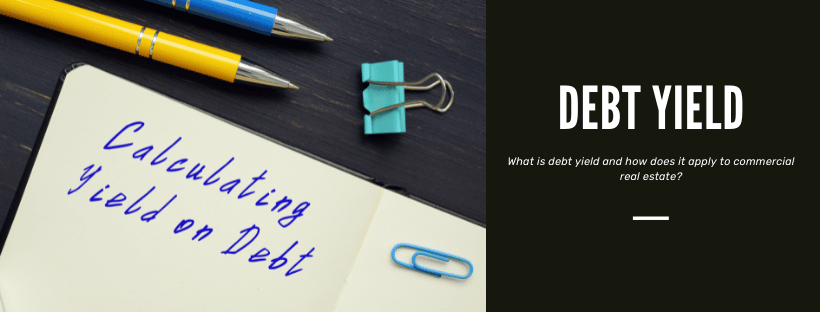The browser version you are using is not recommended for this site.

# Debt Yield Calculator: Here’s How to Calculate Your YieldBy David Luke, Categories CommLoan AcademyDebt yield hasn’t traditionally been a primary commercial real estate loan underwriting metric, but more lenders are incorporating it into their criteria. In the current real estate market, measuring debt yield ratios provides lenders with a stable assessment regardless of unusual or changing conditions.

\$
\$

%

## What is Debt Yield?

Debt yield is a standardized way to measure net operating income (NOI) against total loan value. The ratio is simple to calculate, but it’s an accurate measure of risk that can be used to evaluate individual loans or compare different loans.

### How to Calculate Debt Yield

The math required for a debt yield calculation is simple and easy. The debt yield formula is:

Debt Yield = Net Operating Income / Loan AmountFor example, consider the purchase of a property with \$300,000 NOI and a loan of \$3 million. In this example, the debt yield is 10 percent (\$300,000 / \$3,000,000 = 10%).

Answer a few questions and get custom mortgage quotes. We'll match you with offers from our network of 650+ lenders.

### What Does Debt Yield Tell You?

Lenders use debt yield ratios to determine what their return would be if a buyer immediately defaulted on a commercial real estate loan. Loans with low debt yields are considered riskier, as the lender would receive a smaller return in the event of foreclosure. Higher debt yields are less risky because the lender would receive a larger return and could recoup their losses faster.

Once lenders know what annual return they’d receive, they can calculate how long it’d take to recoup the loss on a foreclosed property. This is done by dividing 100 percent by the debt yield ratio (annual return). The result is the number of years that it’d take to recoup all losses.

Recoup Time = 100 / Debt Yield

Assuming the above debt yield of 12 percent, the lender could recoup their investment in around 8.3 years (100% / 12% = 8.33 years).

Borrowers can alternatively use the ratio to calculate the maximum loan amount a property can qualify for. If the allowed debt yield and net operating income are known, then the loan amount is the NOI divided by the debt yield.

Maximum Loan Amount = Net Operating Income / Debt Yield

If a lender requires a minimum debt yield of 10 percent, the maximum amount that the above example could qualify for would be \$1.2 million (\$120,000 / 0.10 = \$1,200.000).

(Of course, any maximum loan amount would also be subject to loan to value (LTV) and debt service coverage ratio (DSCR) requirements. If a lender considers all three of these ratios, whichever has the lowest permitted loan amount is the one that sets the maximum amount borrowed.)

## How Debt Yield Applies to Commercial Real Estate

Lenders appreciate that debt yield ratio is insulated from variables that can skew loan to values, debt service coverages, and even cap rates. As extremely low-interest rates and spiking property values make accurate loan risk assessment more difficult, debt yield provides a consistent risk measurement when underwriting commercial real estate loans.

### Debt Yield vs. Loan to Value

Loan-to-value ratios depend heavily on the value of a commercial property, and this ratio is susceptible to large swings in property values. Borrowers can potentially get much larger loans when property values increase drastically, and lenders can be underwriting an underwater loan if property values then drop drastically.

In contrast, debt yield ratios aren’t impacted by changes in property value. The loan itself is the underlying denominator, and not how much the property is worth. So long as net operating income doesn’t change, debt yield won’t change after a loan is underwritten.

### Debt Yield vs. Debt Service Coverage

Debt service coverage is based on the annual debt payment, which is affected by interest rate and amortization schedule. Thus, DSCR can be skewed by extreme interest rates (currently extremely low) and/or long amortizations. The DSCR for variable-rate loans will also change as interest rates increase.

Debt yield is based on the loan amount, and thus won’t change with interest rates or amortization schedules. It is thus a more consistent measure in many situations, even though both measurements use net operating income.

### Debt Yield vs. Cap Rate

Although cap rate also looks at net operating income, this is based on the value of a property. Cap rate is thus susceptible to some of the same issues as loan to value is, and which debt yield is insulated against.

## What is an Acceptable Debt Yield?

The Comptroller’s Commercial Real Estate Lending booklet recommends a minimum debt yield of 10 percent, and most lenders that consider this metric follow that recommendation.

In certain situations, lenders may allow a 9 percent debt yield for desirable properties in major markets (e.g. New York City, Los Angeles). Ratios of 8 percent for truly exceptional properties are quite rare, although not altogether unheard of.

Notably, debt yield is based on current net operating income. Projected rent increases or NOI growth isn’t considered when calculating the ratio, so adjusting projections generally won’t have an impact on whether debt yield meets a lender’s minimum requirement.